# Division of Fractions

Division of Fractions
Go back to  'Fractions'

 1 Concept Video on Fraction Division 2 Introduction to Division of Fraction 3 Reciprocal of a Fraction 4 What are the Steps in Dividing Fractions? 5 Divide Fractions by Fractions 6 Divide Fractions with Decimals 7 Divide Fractions with Mixed Numbers 8 Tips and Tricks 9 Solved Examples 10 Practice Questions 11 Challenging Questions 12 Maths Olympiad Sample Papers 13 Frequently Asked Questions (FAQs)

We at Cuemath believe that Math is a life skill. Our Math Experts focus on the “Why” behind the “What.” Students can explore from a huge range of interactive worksheets, visuals, simulations, practice tests, and more to understand a concept in depth.

Book a FREE trial class today! and experience Cuemath's LIVE Online Class with your child.

## Concept Video on Fraction Division- Cuemath Way

Let's learn how to divide fractions the Cuemath way by watching this video.

## Introduction to Division of Fraction

Division means sharing an item equally.

We have learnt about the division of whole numbers.

Similarly, we can divide fractions.

Consider this example.

An apple is divided into two equal parts.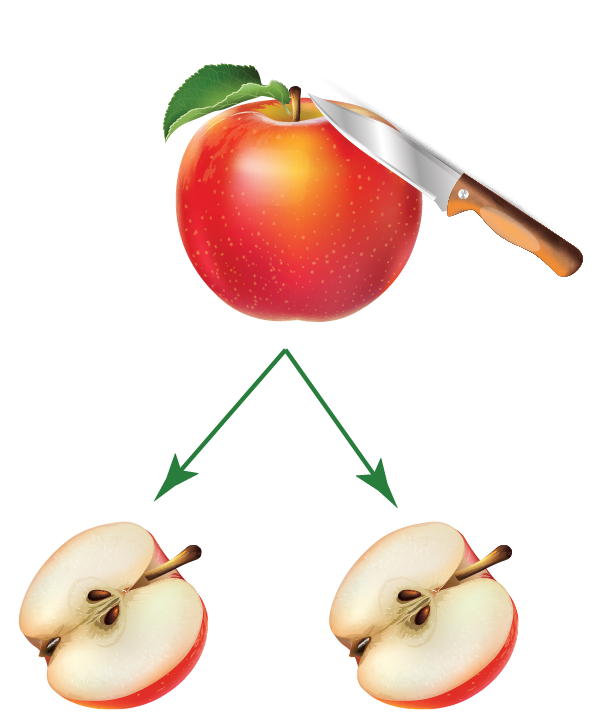As a mathematical statement, we can write it as:

\begin{align}1 \div 2 = \frac{1}{2} \end{align}

Now, let us divide each half apple further into two equal parts.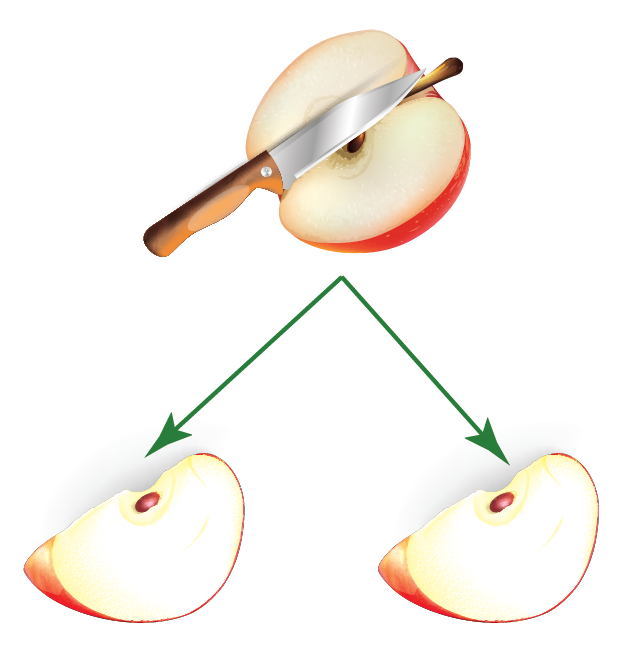As a mathematical statement, we can write it as:

\begin{align}\frac{1}{2} \div 2 = \frac{1}{4} \end{align}

In this chapter, we will learn to:

• Divide fractions by whole numbers
• Divide fractions by fractions
• Divide fractions with decimals
• Divide fractions with mixed numbers

Before learning how to divide fractions, recall how to multiply fractions.

## Reciprocal of a Fraction

Before we start to divide fractions, let us understand what reciprocal means.

Reciprocal of a fraction is another fraction which when multiplied with  the original fraction gives the product as $$1$$

Simply, reverse the numerator and denominator of the given fraction and you get the reciprocal of the given fraction.

Consider the fraction \begin{align} \frac{3}{4}\end{align}

What should we multiply \begin{align} \frac{3}{4}\end{align} with to get $$1$$?

We have to multiply \begin{align} \frac{3}{4}\end{align} with \begin{align}\frac{4}{3}\end{align} to get $$1$$

\begin{equation}
\begin{split}
& = \frac{3}{4}  \times  \frac{4}{3}\\\\
&= 1
\end{split}
\end{equation}

Can you identify the reciprocal of \begin{align}\frac{1}{2}\end{align}?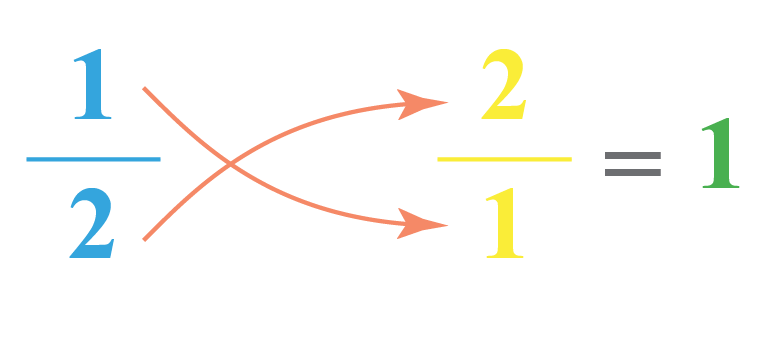## What are the Steps in Dividing Fractions?

We have learnt in whole number division that the dividend is divided by the divisor.

 \begin{align}\text{Dividend} \div \text{Divisor} = \frac{ \text{Dividend}}{\text{Divisor}}\end{align}

We have to see, how many parts of the divisor are there in the Dividend.

Let us consider an example.

1 divided by \begin{align} \frac{1}{6}\end{align}

We need to divide 1 whole by parts of \begin{align} \frac{1}{6}\end{align} and find out how many such parts are needed.

The simulation will help us understand this better.

Use the slider in the below simulation to see how to divide a whole number by a fraction.

We begin placing the first part (green brick/block) and soon realize that we need 6 parts of 1/6 to form 1 whole.

Thus, we get:

\begin{align} 1 \div \frac{1}{6} = 6 \end{align}

When the dividend and the divisor themselves are fractions, this is how we divide the fractions:

• Step 1: Take the reciprocal of the divisor (the second fraction)
• Step 2: Multiply it to the dividend
• Step 3: The product of these two fractions will be the solution.

## Divide Fractions by Fractions

We just learnt how to divide fractions by taking the reciprocal.

Let us see how to divide a fraction by another fraction with an example.

\begin{align} \frac{{{\text{Fraction}}_1 }}{{{\text{Fraction}}_2 }} &= \frac{{\left[ {\frac{{{\text{Numerator}}_1 }\;\left(Nr_1\right)}{{{\text{Denominator}}_1 }\;\left(Dr_1\right)}} \right]}}{{\left[ {\frac{{{\text{Numerator}}_2 }\;\left(Nr_2\right)}{{{\text{Denominator}}_2 }\;\left(Dr_2\right)}} \right]}} \\\\ & = \frac{{{\text{Nr}}_1 }}{{{\text{Dr}}_1 }} \times \frac{{{\text{Dr}}_2 }}{{{\text{Nr}}_2 }} \\ \end{align}

Consider the following example:

\begin{align} \frac{ (\frac{1}{2})}{(\frac{3}{4})} &=\frac{1}{2} \div \frac{3}{4} \\\\ &= \frac{1}{2} \times \frac{4}{3} \\\\ &=\frac{4}{6}\\\\ &=\frac{2}{3} \end{align}

## Divide Fractions with Decimals

We know that decimal numbers themselves are a fraction to base $$10$$

Example:

\begin{align}1.5 = \frac{15}{10} ;\; 0.2 = \frac{2}{10}\end{align}

We can represent the decimal in the fractional form and then perform the division.

Consider the example

\begin{align} \frac{\frac{3}{4}}{0.5} &=\frac{ \frac{3}{4}}{\frac{5}{10}} \;( \because 0.5 \!=\!\frac{5}{10}) \\\\ &= \frac{3}{4} \times \frac{10}{5} \\\\ &=\frac{30}{20}\\\\ &=\frac{3}{2} \end{align}

## Divide Fractions with Mixed Numbers

We have learnt how to convert mixed fraction to improper form.

To divide fractions with mixed form, we have to convert the mixed fraction to improper form and then divide them.

Consider the following example.

\begin{align}\frac{ \frac{3}{4}}{1\frac{1}{2}} \end{align}

This can be solved in the following way:

\begin{align} \frac{\frac{3}{4}}{1\frac{1}{2}} &=\frac{ \frac{3}{4}}{\frac{3}{2}} \;( \because 1\frac{1}{2}\!=\!\frac{(2\!\times\!1) \!+\! 1}{2} \!=\!\frac{3}{2} )\\\\ &= \frac{3}{4} \times \frac{2}{3} \\\\ &=\frac{1}{2} \end{align}

Tips and Tricks
1. When dividing fractions, see how many $$\frac{\text{c}}{\text{d}}$$ are contained in $$\frac{\text{a}}{\text{b}}$$, and visualise \begin{align}\frac{\frac{\text{a}}{\text{b}}}{\frac{\text{c}}{\text{d}}}\end{align} as \begin{align}\frac{\text{a}}{\text{b}} \!\div\! \frac{\text{c}}{\text{d}}\end{align}
2. Remember this easy rhyme when dividing two fractions:

🎜When you want to divide two fractions,
Take the reciprocal of the second one,
Multiply it with the first one,
Voila, you have divided the given fractions!
🎝

Help your child score higher with Cuemath’s proprietary FREE Diagnostic Test. Get access to detailed reports, customized learning plans, and a FREE counseling session. Attempt the test now.

## Solved Examples

 Example 1

Solve

\begin{align}1\frac{1}{2} \div \frac{3}{2}\end{align}

Solution:

\begin{align} \frac{1\frac{1}{2}}{\frac{3}{2}} &=\frac{ \frac{3}{2}}{\frac{3}{2}} \;(\because 1\frac{1}{2} \!=\!\frac{(2 \!\times\!1) \!+\! 1}{2} \!=\!\frac{3}{2} )\\\\ &= \frac{3}{2} \times \frac{2}{3} \\\\ &=1 \end{align}

 $$\therefore$$\begin{align} 1\frac{1}{2} \div \frac{3}{2} = 1\end{align}
 Example 2

Solve

$$0.5 \;\div$$ \begin{align} \frac{3}{4}\end{align}

Solution:

\begin{align} 0.5 \div \frac{3}{4} &= \frac{5}{10} \div \frac{3}{4} \;(\because0.5= \frac{5}{10}) \\\\ &= \frac{5}{10} \times \frac{4}{3} \\\\ &=\frac{20}{30} \\\\ &= \frac{2}{3} \end{align}

 $$\therefore$$ \begin{align} 0.5 \div \frac{3}{4} = \frac{2}{3}\end{align}
 Example 3

John has $$4$$ pizzas to share with his friends.

The pizzas are cut into equal slices and each person eats \begin{align}\frac{1}{8}\end{align} of the pizza.

How many friends can he share the pizzas with?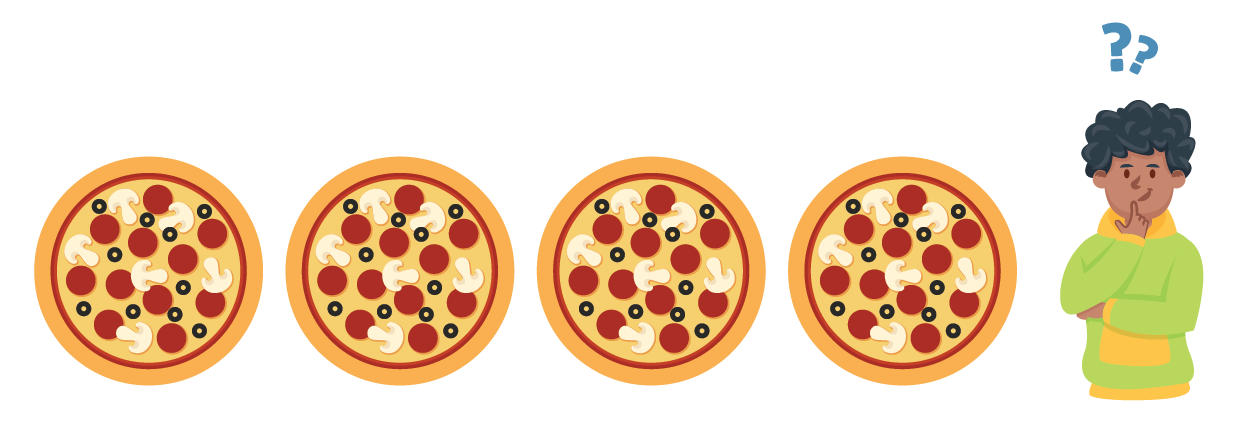Solution:

Total number of slices of pizza with John are:

\begin{align} 4 \div \frac{1}{8} &=4 \times \frac{8}{1} \\\\ &= 32 \end{align}

 $$\therefore 32$$ friends can share $$4$$ pizzas.
 Example 4

There are \begin{align}\frac{1}{60}\end{align} minutes in $$1$$ second.

Calculate the number of seconds in \begin{align}\frac{1}{10}^{th}\end{align} of a minute.

Solution:

In \begin{align}\frac{1}{10}^{th}\end{align} of a minute, there are:

\begin{align} &= \frac{\frac{1}{10}}{\frac{1}{60}}\: \text{seconds} \\\\ &= \frac{1}{10} \times \frac{60}{1} \\\\ &= \frac{60}{10} \\\\ &=6 \:\text{seconds} \end{align}

 $$\therefore$$\begin{align}\frac{1}{10}^{th}\end{align} of a minute has $$6$$ seconds
 Example 5

Tim has $$1\frac{1}{2}$$ L of juice in a jug.

He has to pour the juice into cups.

Each cup can hold $$\frac{1}{4}$$ L of juice.

How many cups will he need to pour all the juice?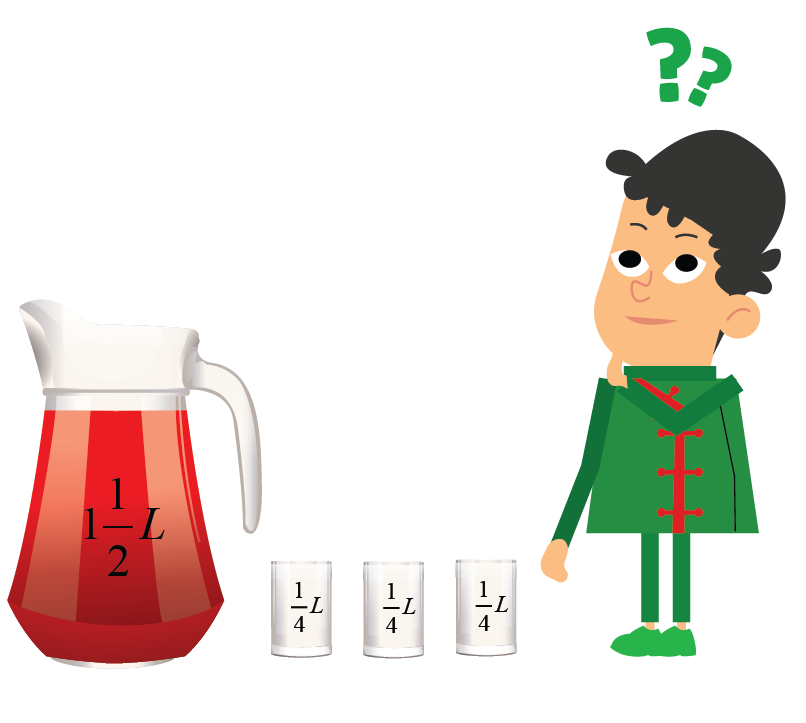Solution:

Number of cups needed:

\begin{align} &= \frac{1\frac{1}{2}}{\frac{1}{4}} \\\\ &= \frac{\frac{3}{2}}{\frac{1}{4}} \;(\because 1\frac{1}{2} = \frac{3}{2})\\\\ &= \frac{3}{2} \times \frac{4}{1} \\\\ &= 6 \end{align}$$\therefore$$Tim needs $$6$$ cups.

CLUEless in Math? Check out how CUEMATH Teachers will explain Division of Fractions to your kid using interactive simulations & worksheets so they never have to memorise anything in Math again!

Explore Cuemath Live, Interactive & Personalised Online Classes to make your kid a Math Expert. Book a FREE trial class today!

## Practice Questions

Here are a few activities for you to practice. Select/Type your answer and click the "Check Answer" button to see the result.Challenging Questions
1. By what number should $$\frac{2}{5}$$ be multiplied to get $$3 \frac{1}{2}$$?
2. Julie has $$5\frac{2}{5}$$ m long ribbon. She needs to cut ribbon strips of length $$0.6$$ m long. How many ribbon strips can she cut?
3. Amy walks $$2\frac{1}{7}$$ km in $$1$$ hour  $$15$$ minutes. How long can she walk in     $$30$$ minutes?

IMO (International Maths Olympiad) is a competitive exam in Mathematics conducted annually for school students. It encourages children to develop their math solving skills from a competition perspective.

## 1. What are the steps in dividing fractions?

Follow these steps to divide fractions:

• Take the reciprocal of the second fraction
• Multiply it with the first fraction

\begin{align} \frac{{{\text{Fraction}}_1 }}{{{\text{Fraction}}_2 }} &= \frac{{\left[ {\frac{{{\text{Numerator}}_1 }\;\left(Nr_1\right)}{{{\text{Denominator}}_1 }\;\left(Dr_1\right)}} \right]}}{{\left[ {\frac{{{\text{Numerator}}_2 }\;\left(Nr_2\right)}{{{\text{Denominator}}_2 }\;\left(Dr_2\right)}} \right]}} \\\\ & = \frac{{{\text{Nr}}_1 }}{{{\text{Dr}}_1 }} \times \frac{{{\text{Dr}}_2 }}{{{\text{Nr}}_2 }} \\ \end{align}

## 2. What are the ways to teach dividing fractions?

Divison can be taught in many ways such as, by using models or applying the concept of multiplication of fractions.

A simple way to explain how to divide fractions is :

1. Flip the reciprocal of the divisor.
3. Multiply it with the dividend.

## 3. How can you divide a number by a fraction?

• To divide a whole number by a fraction, we will multiply the whole number with the reciprocal of the fraction.

\begin{align} \frac{ 5}{\frac{2}{3}} &=5 \div \frac{2}{3} \\ &= 5 \times \frac{3}{2} \\ &=\frac{15}{2} \end{align}

• To divide a fraction by a fraction, we will multiply the reciprocal of the second number with the first number.

\begin{align} \frac{ \frac{3}{5}}{\frac{3}{4}} &=\frac{3}{5} \div \frac{3}{4} \\ &= \frac{3}{5} \times \frac{4}{3} \\ &=\frac{4}{5} \end{align}

More Important Topics
Numbers
Algebra
Geometry
Measurement
Money
Data
Trigonometry
Calculus
More Important Topics
Numbers
Algebra
Geometry
Measurement
Money
Data
Trigonometry
Calculus
Learn from the best math teachers and top your exams

• Live one on one classroom and doubt clearing
• Practice worksheets in and after class for conceptual clarity
• Personalized curriculum to keep up with school